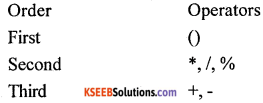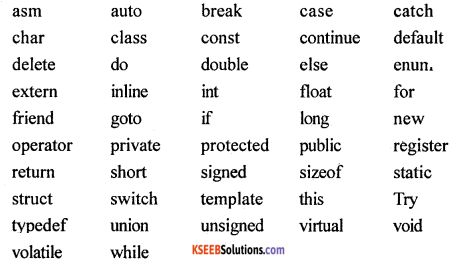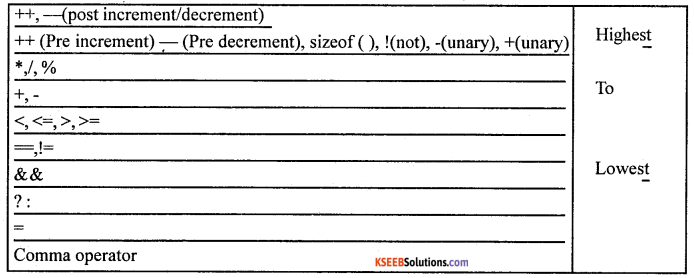# 1st PUC Computer Science Question Bank Chapter 7 Introduction to C++

## Karnataka 1st PUC Computer Science Question Bank Chapter 7 Introduction to C++

### 1st PUC Computer Science Introduction to C++ One Mark Questions and Answers

Question 1.
Who is the creator of the C++ programming language?
The creator of C++ is Bjarne Stroustrup.

Question 2.
What is C++?
C++is an object-oriented programming language.

Question 3.
What was the earlier name of C++?
The earlier name of C++ was ‘C with classes’.

Question 4.
Who gave the name ‘C++’?
The name C++ was given by Rick Mascitti.

Question 5.
Name any two characteristics of C++.
Object-oriented programming and portability are two characteristics of C++.

Question 6.
Describe tokens.
The smallest individual units in a program are known as tokens, on lexical units.

Question 7.
Mention a few tokens.
A few tokens are keywords, identifiers, constants, strings, and operators.

Question 8.
What is a keyword?
All keywords (reserved words) are basically the sequences of characters that have one or more fixed meanings.

Question 9.
What are identifiers?
Identifiers are names given to the program elements such as variables, arrays, and functions.Question 10.
What are constants?
The program elements, which not change during the execution of a program, are known as constants.

Question 11.
Define a character set.
It is a set of symbols that a programming language identifies and is used in writing data and instructions in a programming language.

Question 12.
What is an integer constant?
Integer constants are whole numbers without any fractional part.

Question 13.
What are floating-point constants?
Floating-point constants are numeric values that contain a decimal point and can also contain exponents.

Question 14.
Define octal constant.
It consists of a sequence of digits starting with 0 (zero).

Question 15.
It consists of a sequence of digits from 0 to 9 and A, B, C, D, E, F alphabet symbols that represent decimal numbers 10, 11, 12, 13,14, and 15 respectively, preceded by ox or OX.

Question 16.
What are the character constants?
A character constant in C++ must contain one or more characters and must be enclosed in single quotation marks.

Question 17.
What are punctuators?
Punctuators are symbols other than alphabets and numbers that are used in C++.

Question 18.
Define string constants.
A sequence of characters enclosed within double quotes is called a string constant or literal.Question 19.
What is the operator?
An operator is a symbol that tells the compiler to performs specific operations and give a value as the result.

Question 20.
What are the arithmetic operators?
The symbols that perform addition, subtraction, multiplication, modulus, and division are called arithmetic operators.

Question 21.
Give the difference between / and % arithmetic operators.

• The arithmetic operator / performs division and gives out the quotient as a result.
• The arithmetic operator % performs division and gives out the remainder as a result.

Question 22.
What is an operand?
The operand is a data item on which the operator performs some activity.

Question 23.
What are unary operators?
An operator that takes only one operand to perform some operation is called a unary operator.

Question 24.
What are binary operators?
The operators which take two operands to perform some operation is called a binary operator.

Question 25.
What are the relational operators?
The operators which perform an operation of the relation between two operands are called relational operators.

Question 26.
What are the logical operators?
The operator which perform combine or negate the expressions that contain relational operators are called logical operators.

Question 27.
What is the function of the bitwise operator?
Bit manipulation operators manipulate individual bits within a variable. Bitwise operators modify variables considering the bit patterns that represent the values they store.

Question 28.
What is meant by shorthands in C++?
In C++ short hands means writing certain types of assignment statements in a simplified manner.Question 29.
What is a ternary operator?
The operator that operates on three or more operands is called a ternary operator.

Question 30.
What is an expression?
An expression is a combination of constants, variables, operators and function calls which produces a particular value to be used in some other context.

Question 31.
What is a statement?
The statement is an instruction to the computer telling it what to do for instance assigning an expression value to a variable because it produces an instruction tells the computer to assign a value to something.

Question 32.
Give an example for assignment operators.
Example for assignment operators is a = 20;

Question 33.
Give an example for sizeof operator.
If k is integer variable, the size of (k) returns 2.

Question 34.
What is operator precedence?
The order in which the arithmetic operators (+,-,*,/,%) are used in a given expression is called the order of precedence.Question 35.
What is typecast?
Typecasting is making a variable of one type, such as an int act like another type, a char, for anyone single operation.

Question 36.
Mention the types of typecasting.
The two types of typecasting are implicit conversion and explicit conversion.

Question 37.
What is the use of iostream.h in C++?
iostream stands for input/output stream. It is a header file which we include in our programs, to perform basic input-output operations.

Question 38.
Mention any two ctype.h functions.
Toupper() and tolower() are the two examples for ctype.h functions.

Question 39.
Mention any two string.h functions.
Strupr() and strlwr()

Question 40.
Mention any two functions of stdio.h
Printf() and scanf() are two functions of stdio.h

Question 41.
Mention any two functions of stdlib.h
Atoi() and itoa() are two functions for stdlib.h

Question 42.
What is a preprocessor directive?
A preprocessor directive is a command that is considered for execution before the processor executes the program.

### 1st PUC Computer Science Introduction to C++ Two/Three Marks Questions and Answers

Question 1.
What is C++?
C++ is an object-oriented programming language. It is also known as C with classes. It is a combination of C and Simula 67.
It was invented by Bjarne Stroustrup at AT &T Bell Lab in New Jersey, USA. in the early 1980’s.

Question 2.
Write any two characteristics of C++.
Object-oriented programming and portability are important two characteristics of C++.Question 3.
What is meant by a character set? Give an example.
Character set is a set of valid characters that a language can recognize.

• Letters A – Z, a – z
• Digits 0 – 9
• Special Characters + – * / ˆ \ () [] {} = != <> ‘ “ \$ , ; : % ! & ? _ # <= >= @

Question 4.
What are the different tokens available in C++?
A token is a group of characters that logically belong together. C++ uses the following types of tokens. Keywords, Identifiers, Literals, Punctuators, and Operators.

Question 5.
What is the function of the keywords? Write any four keywords.
These are some reserved words in C++ which have a predefined meaning to the compiler, called keywords. Some commonly used keywords are:
int, float, char, and case

Question 6.
Write any two rules for naming the identifier.
Two rules for the formation of an identifier are that it can consist of alphabets, digits and/or underscores and it must not start with a digit.

Question 7.
Mention different types of literals available in C++.
The following types of literals are available in C++.

• Integer-constants
• Character-constants
• Floating-constants
• Strings-constants

Question 8.
Write any four punctuators of C++.
Four punctuator of C++are (),{}, ; , and :

Question 9.
How many types of operators are available in C++? Mention any two.
C++ provides six types of operators. Arithmetical operators and Relational operators are two such operators.Question 10.
Write the use of arithmetical operators.
Arithmetical operators +, -, *, /, and % are used to perform an arithmetic (numeric) operation.

Question 11.
Why is a relational operator used?
Relational operators are used to test the relation between two values. A relational expression returns zero when the relation is false and a non-zero when it is true.

Question 12.
Why are logical operators are used? Give an example.
Logical operators are used to combine one or more relational expressions. Examples of logical operators are || (OR), && (AND).

Question 13.
How many unary operators are available in C++? Give an example.
C++ provides two unary operators for which only one variable is required.
For Example a = – 50;

Question 14.
Explain a ternary operator with an example.
The operator which uses three or more expressions is called a ternary operator. It is also called a conditional operator. For example, conditional Expression? expression1: expression2; if the conditional Expression is true, expression 1 executes, otherwise if the conditional Expression is false, expression 2 executes.

Question 15.
Explain shorthand operators with an example.
In C++ shorthand operators mean writing certain type of assignment statements in a simplified manner. For example, if x = 5 and x + = 10 means x = x +10 i.e., x = 5 + 10 = 15.

Question 16.
Mention different bitwise operators.Question 17.
Mention any two special operators and their meaning.
Sizeof() – it returns the size of a variable.
. (dot) – member operator used to reference member of a structure.Question 18.
What is the use of assignment operator? Give an example.
The assignment operator ‘=’ is used for assigning a variable to a value. This operator takes the expression on its right-hand-side and places it into the variable on its left-hand-side.
For example: m = 5;

Question 19.
What do you mean by the precedence of operators? Give an example.
The order in which the Arithmetic operators (+,-,*,/,%) are used in a given expression is called the order of precedence. The following table shows the order of precedence.Question 20.
What is implicit conversion? Give an example.
When two operands of different types are encountered in the same expression, the lower type variable is converted to the higher type variable which is called implicit conversion.
For example, float = int + float

Question 21.
Explain the use of explicit conversion with an example.
This is also called as typecasting. It temporarily changes a variable data type from its declared data type to a new one.
For example, T_Pay = double (salary) + bonus;

Question 22.
Write a simple C++program.
#include
main()
{
cout<< “Hello World”; // prints Hello World
return 0;
}

Question 23.
How are comments included in C++? Give an example.
For example,
main()
{
cout<< “Hello World”; // prints Hello World
return 0;
}

### 1st PUC Computer Science Introduction to C++ Five Marks Questions and Answers

Question 1.
Write a note on C++.

• C++ is a typed, compiled, general-purpose, case-sensitive, free-form programming language that supports procedural, object-oriented, and generic programming.
• C++ is regarded as a middle-level language, as it comprises a combination of both high-level and low-level language features.
• C++ was developed by Bjarne Stroustrup starting in 1979 at Bell Labs in Murray Hill, New Jersey as an enhancement to the C language and was originally named C with Classes but later it was renamed C++ in 1983. by Rick Masatti.
• C++ is a superset of C, and virtually, any legal C program is a legal C++ program.

Question 2.
Write the applications of C++.

• It is a versatile language for handling very large programs
• It is suitable for virtually any programming task, including development of editors, compilers, databases, communication systems and any complex real life application systems
• It allows us to create hierarchy-related objects, so we can build special object-oriented libraries which can be used later by other programmers.
• While C++ is able to map the real-world problem properly, the C part of C++ gives the language the ability to get close to the machine-level details.
• C++ programs are easily maintainable and expandable.

Question 3.
Write a short note on the keywords of C++.
These are some reserved words in C++ which have some predefined meanings to the compiler and are called keywords. Some commonly used Keywords are given below:Question 4.
Write the rules to be followed, while naming the identifier.
The identifier is a sequence of characters taken from the C++ character set. The rules for the formation of an identifier are:

• It can consist of alphabets, digits and/or underscores.
• C++ is case sensitive, that is upper and lower case letters are considered as different from each other.
• It should not be a reserved keyword.

Question 5.
Write a short note on literals.
The five arithmetical operations supported by the C++ language are:
– subtraction
* multiplication
/ division
% modulus
Operations of addition, subtraction, multiplication, and division literally correspond with their respective mathematical operators.
For example,
sum = 5 + 10;
difference = 10 – 5;
product = 2 * 5;
quotient = 10 / 2;
remainder = 11 % 3;Question 6.
Write a short note on the precedence of operators.
The following table shows the order of precedence.The following table shows the precedence of operators.Question 7.
Explain the Structure of a C++ program (with an example)
#include
// main()
int main()
{
cout<< “Hello World”; // prints Hello World
return 0;
}
The various parts of the above program:

• Headers, which contain information that is either necessary or useful to the program. For this program, the header is needed.
• The next line // main() is where the program execution begins. It is a single-line comment available in C++. Single-line comments begin with // and stop at the end of the line.
• The line int main() is the main function where program execution begins.
• The pair of {} indicates the body of the main function.
• The next line cout<< “Hello World.”; causes the message “Hello World” to be displayed on the screen.
• The next line return 0; terminates main( )function and causes it to return the value 0 to the calling process.
error: Content is protected !!